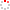#CodeStepByStep## findLongestPath

Language/Type: C++ Graph collections

Write a function named `findLongestPath` that accepts as a parameter a reference to a `BasicGraph`, and returns a `Vector` of strings representing the names of the vertexes in the longest possible "simple" path between any two vertexes in that graph. A simple path is a non-cycle path that does not repeat any vertexes. Your algorithm does not consider edge weight/cost, only path length.

The diagram below shows an example graph that might be passed to your algorithm. In the following graph, the longest possible simple path is {D, G, H, F, A, B, C}, which has length 7. So when passed the graph below, your function would return a vector containing the names of those vertexes in that order. If there is a tie and there are multiple longest paths of exactly the same length, your function can return any one of those equally longest paths. If the graph does not contain any vertexes, your function should return an empty vector. If the graph does contain vertexes but does not contain any edges, your function should return a one-element vector containing any single vertex.

Graph 1:You have learned and written several algorithms for efficiently finding shortest paths and minimum-weight paths. It turns out that the task of finding longest paths, like you're doing in this problem, doesn't have a known clever algorithm. You need to literally try generating all possible simple paths in the graph and discover which one is the longest through brute force. So you should come up with an algorithm that can enumerate every valid simple path in the graph and find the longest such one. Note that the graph might be cyclic, as in the graph above that contains cycles such as {A, D, G, H, F, A}.

Although you must try every possible path, you should still write code that is efficient. You should not explore large numbers of paths and possibilities multiple times, or continue exploring paths that are certain to be dead-ends.

Constraints: You may assume that the graph's state is valid, and that it is directed and unweighted, and that contains no self-edges (e.g. from V1 to V1). You may define private helper functions if so desired, and you may construct auxiliary collections as needed to solve this problem. You should not modify the contents of the graph such as by adding or removing vertexes or edges from the graph, though you may modify the state variables inside individual vertexes/edges such as `visited`, `cost`, etc.

Type your C++ solution code here:

This is a function exercise. Write a C++ function as described. Do not write a complete program; just the function(s) above.